### Why should I choose AnalystNotes?

AnalystNotes specializes in helping candidates pass. Period.

##### Subject 7. The Standard Normal Distribution
The problem with working with a normal distribution is that its formula is very complicated. A computer is needed to calculate areas under the graph; this is required in order to calculate probabilities.

The way to get around this problem is to standardize a normal random variable, which involves converting it to a general scale for which probability tables exist.

The standard normal distribution is a normal distribution with a mean of 0 and a standard deviation of 1. It is denoted as N(0,1). Below are some confidence intervals for the standard normal distribution: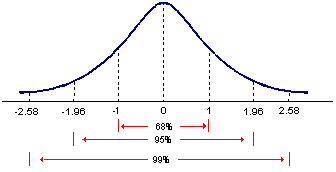There is an unlimited number of normal distributions, each with a different mean or standard deviation. Therefore, it's impractical to provide a table of probabilities for each combination of mean and standard deviations. However, the actual distribution for a normal random variable to a standard normal distribution can be standardized. Normal distributions can be transformed to standard normal distributions by the formula:where X is a score from the original normal distribution, μ is the mean of the original normal distribution, and σ is the standard deviation of original normal distribution.

The standard normal distribution is sometimes called the z distribution. A z score (also called z-value or z-statistic) is the distance between a selected value (X) and the population mean, divided by the population standard deviation. It is in fact a standard normal random variable. For instance, if a person scored 70 on a test with a mean of 50 and a standard deviation of 10, that person scored 2 standard deviations above the mean. Converting the test scores to z scores, an X of 70 would be: z = (70 - 50) / 10 = 2.

So, a z score of 2 means the original score was 2 standard deviations above the mean. Note that the z distribution will only be a normal distribution if the original distribution (X) is normal.

Example

The rates of return on the assets in a portfolio are normally distributed, with a mean of 20% and a standard deviation of 12%. What's the probability that the return on an asset falls between -3.52% and 50.96%?

Solution:

• If the return on the asset is -3.52%, its z-value is z = (-3.52% - 20%)/12% = -1.96.
• If the return on the asset is 50.96%, its z-value is (50.96% - 20%)/12% = 2.58.
• Recall that a normal distribution is symmetrical. The 95% confidence interval is -1.96 to 1.96. The 99% confidence interval is -2.58 to 2.58. The probability of z-value falling between -1.96 and 2.58 is 95%/2 + 99%/2 = 97%.

How to Use the Z-Table

The z-table gives cumulative probability values for a Z-graph (or standard normal distribution). In other words, by looking up a particular z-value, the probability of all values smaller than this value can be found.

Recall that a cumulative distribution function gives probabilities of the form: P (X < x), that is, probabilities that are less than the value being examined.
In the same way, the table gives probabilities of the form: P(Z < z), that is, probabilities that are less than the z-value being looked up or calculated.

The first column, headed z, ranges from 0.0 to 4.0. Across the top, the values range from 0.00 to 0.09. These values allow a person to look up any z-value to two decimal places. The vertical column gives the value to one decimal place and the horizontal row gives the values in the second decimal position. So, for example, if you want to look up z = 0.26, you would go to the row that starts 0.2 and go to the column headed 0.06. You can then read the value 0.6026, which means that 60.26% of z-values or z-scores are less than 0.26.

So, the body of the table (the middle part) gives probabilities (or areas under the graph); hence; all the values in the body of the table lie between 0 and 1. Actually, they lie between 0.5 and 1, because the table starts considering z-values from the point 0.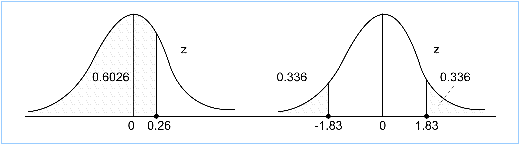You can see that 60.26% of the area under the graph lies to the left of the point z = 0.26. Therefore, 39.74% of the area lies to the right of this point. Because areas under continuous graphs give probabilities, you can also say that: P(Z < 0.26) = 0.6026.

At this point, you might have a question. Z-scores generally range from -4 to 4, but the table has no negative values. Why not?

To answer this, recall that a normal distribution is symmetrical about its mean; this fact can be used to calculate probabilities for negative z-values very easily.

Example

Take the point z = 1.83. The table shows the area to the left of 1.83 as 0.9664. The area to the right of 1.83 is therefore 1- 0.9664 = 0.0336. Because of symmetry, the area to the right of 1.83 is the same as the area to the left of -1.83. So, if the area to the left of a negative value is required, simply take 1 - the area to the left of the equivalent positive value. Problem solved.

Continuing with this example, the area to the left of -1.83 is 0.0336, which is the probability of obtaining a z-score smaller than -1.83.

A portion of the z-table is presented below. (N(z) represents the cumulative probability distribution for a standard random variable Z):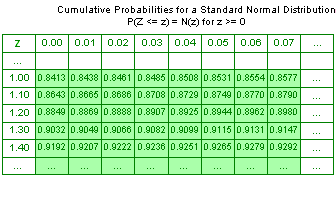Example

Suppose that you want to find the probability that a standard normal variable is less than or equal to 1.01. In the z-table, go down the column headed by the letter z to 1.00. Move to the right and read the entry under the column headed 0.01. It's 0.8438. That is, P(Z ≤ 1.01) = 0.8438 or 84.38%, and P(Z ≥ 1.01) = 15.62%.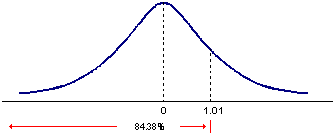A standard normal distribution is symmetrical with a mean of 0. Therefore, P(Z ≤ 0) = 50% ==> P(0 ≤ Z ≤ 1.01) = 0.8438 - 0.5 = 34.38%.

Comprehensive Example

The heights of people in a city are normally distributed with mean 170 cm and standard deviation 10 cm. Calculate the probability that a randomly chosen person from this population has a height that is:

• less than 180 cm
• less than 150 cm
• greater than 175 cm
• between 160 cm and 185 cm
• greater than 150 cm

Because there is a normal distribution that is not standardized, it is important to standardize each value before looking up answers in a z-table. Let X = the heights of people in the population.

1. You want P(X < 180). Standardizing gives P(Z < (180-170)/10) = P(Z < 1). From tables, look up 1.00 and you get 0.8413. So, the answer is 0.8413.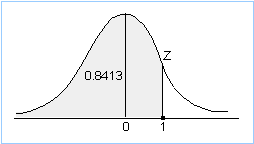2. You want P(X < 150). Standardizing gives P(Z < (150-170)/10) = P(Z < -2). Recall that this probability is the same as P(Z > 2), due to the symmetrical nature of the normal distribution. From tables, look up 2.00 and you get 0.9772. This is the probability that Z<2, so 1 - 0.9772 = 0.0228 is the probability that we want. So, the answer is 0.0228.3. You want P(X > 175). Standardizing gives P(Z > (175-170)/10) = P(Z > 0.5). From tables, look up 0.50 and you get 0.6915. This is the probability that Z < 0.5, so 1-0.6915 = 0.3085 is the probability that we want. So, the answer is 0.3085.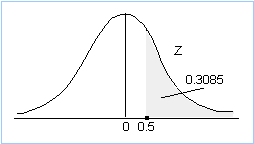4. You want P(160 < X < 185). Standardizing gives P(-1 < Z < 1.5). In order to find a region like this, note that this region can be written as P(Z < 1.5) - P(Z < -1). From tables, look up 1.50 and you get 0.9332. This is the probability that Z < 1.5. To find P(Z < -1), look up 1.00 and you get 0.8413. 1 - 0.8413 = 0.1587 is therefore the probability you want. So, the answer is 0.9332 - 0.1587 = 0.7745.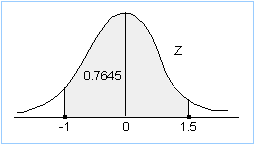5. You want P(X > 150). Standardizing gives P(Z > (150-170)/10) = P(Z > -2). Using symmetry, P(Z > -2) = P(Z < 2). From tables, look up 2.00 and you get 0.9772. So, the answer is 0.9772.Notes:

• These examples encompass the different types of regions you can get. Familiarize yourself with how each region works. If you can do these five examples, you know that you understand this process well.
• Always standardize before going to tables.
• As a check, if the region you are looking for is bigger than half the area under the graph, the answer will be bigger than 0.5 and vice versa. Verify this factor to make sure that you haven't made a silly mistake.
• It is possible to work in reverse. For example, a standardized value can be converted back to an X value using X = σZ + μ. So, in the height example above, a standardized value of 3 is equivalent to an X value of 10 x 3 + 170 = 200 cm.

Learning Outcome Statements

explain how to standardize a random variable;

calculate and interpret probabilities using the standard normal distribution;

CFA® 2023 Level I Curriculum, Volume 1, Module 4

User Comment
vishu100 Extremely well presented and concise
aravinda I second that
ofabian this clears everything up - thanks.
DonAnd Made everything so much clearer.
zeppelin Seriously, this site is great. I'll be using it again for sure.
shivendu It seriously amazing!!!!!!!
Raok haha definitely to the point. just make sure you read the equation to find X value, very important concept
dhess0 The "tables" in the above comprehensive example will be given? Correct? Or did I miss something.
NikolaZ you're not expected to memorize the z table dhess0, so yes it will either be given or the z-score will be provided and you may be required to work backwards
johntan1979 Wow! The example of the -3.52% and 50.96% is really good stuff! Never knew it could be 1.96 on one end and 2.58 on the other end. Thanks AnalystNotes!
sharky7 I finally managed to understand this stuff!!!
schweitzdm I didn't like the basic questions for this section. Need to place the tables in the question.
mvross Great explanation
guest yup great explaining. Now I feel like a dizzy goose, need to take a break from this crap.
JCarney Much better explained than the CFA books.
MathLoser I love it!!!